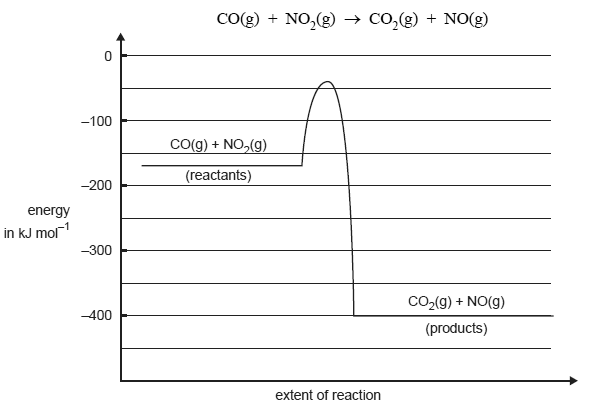Thermochemistry (2005 VCE) The graph below represents the energy changes over the course of a chemical reactionGive the magnitude and sign of the ΔH for the forward reaction in kJ/mol. Solution Give the activation energy for the reverse reaction in kJ mol1. Solution Give two reasons explaining why the rate of this reaction increases with increasing temperature. SolutionA suitable catalyst is discovered for the reaction. What would be the likely effect of the catalyst on: - the activation energy? Explain your answer. Solution - the ΔH? Explain your answer. Solution